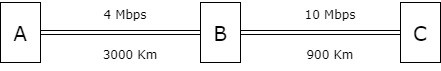## 1. Introduction

In terms of networking, a packet can be defined as a unit of data that moves from source to destination. Data delivered across computer networks such as the Internet is separated into packets. The computer or device that receives them recombines these packets.

Further, the delay or latency can be defined as the time taken by the entire message/packet to reach its destination after the initial bit is sent out from the source. It is an important metric to measure network performance. The delay of a packet is calculated by adding the following four components: propagation delay, transmission delay, queuing delay, and processing delay.

This article discusses propagation delay and transmission delay as these are harder to calculate than the other two delays.

## 2. Propagation Delay

The propagation delay is the time it takes for one bit to travel from one end of the link to the other. The bits travel in the form of electromagnetic signals. The speed at which electromagnetic signals propagate is determined by the medium through which they pass. Following is the formula for propagation delay:whereis the distance between sender and receiver over a link, andis the transmission speed.

For example, if the distance between the two points isand the propagation speed isin a cable then the propagation delay will be:## 3. Transmission Delay

Transmission delay is the time needed to push all the packet bits on the transmission link. It mainly depends upon the size of the data and channel bandwidth (in bps). Following is the formula for transmission delay:whereis the length of the packet andis the transmission rate.

For example, the transmission of() using a transmission rate ofwill take:## 4. Real Life Problems

To better understand the concept, this section explains three different kinds of problems related to propagation and transmission delays and methods to solve such problems.

### 4.1. Problem 1

Let’s see how we can calculate the latency (total delay from first bit sent to last bit received) for the following scenarios.

First, the sender and receiver are separated by twolinks and a single switch as shown in the figure below. The packet size is, and each link introduces a propagation delay of. Let’s assume that the switch begins forwarding immediately after it has received the last bit of the packet and the queues are empty:For each link, the time to transmit the packet on the link is, after which it takes an additionalfor the last bit to propagate across the link. Thus, with only one switch that starts forwarding only after receiving the whole packet, the total transfer delay is two transmission delays and two propagation delays:.

Now, let’s see another scenario with three switches and four links, as shown in the figure below, what will be the delay?For three switches and thus four links, the total delay is four transmission delays and four propagation delays:.

### 4.2. Problem 2

Next, let’s see to another problem where two hosts are connected via a packet switch withlinks. Each link has a propagation delay of. The switch begins forwarding a packetafter receiving it. Ifbits of data are to be transmitted between the two hosts using a packet size ofbits, calculate the time elapsed between the transmission of the first bit of data and the reception of the last bit of the data in microseconds:Sender host transmits the first packet to the switch, the transmission time iswhich is. After, the second packet is transmitted. Therefore, the first packet reaches the destination in.

While the first packet is traveling to the destination, the second packet starts its journey after. The rest of the time taken by the second packet overlaps with the first packet. Eventually, the overall time is.

### 4.3. Problem 3

As a last practice problem, we’ll look at a scenario depicted by the following diagram wherewants to send a 5 MB file toin chunks of 500-byte packets. To prevent any packet loss, whensends a 500-byte packet to, it responds with a 50-byte packet to(through) acknowledging that it has successfully received the packet. Only after receiving the acknowledgment,sends the next packet. Assuming no losses, how long will it taketo send the file to:First, we’ll find the delay for the packet size of 500 bytes fromto.

• Transmission delay fromto:.
• Transmission delay fromto:.
• Propagation delay fromto:.
• Propagation delay fromto:.

Delay on link is the transmission delay and the propagation delay. Hence, the delay betweentois. The delay fromtois. The total delay for packet size of 500 bytes fromtoisNext, let’s find the delay for the acknowledgment packet size of 50 bytes fromto. Propagation delay would remain the same.

• Transmission delay fromto:.
• Transmission delay fromto:.

Hence, the delay betweentoisand the delay fromtois.

• The total delay for acknowledgement fromtois.
• The total delay for packet and acknowledgement is.
• Number of packets in 5MB file transfer is.

Eventually, the total transfer time for a 5MB file is## 5. Conclusion

In this article, we have explained the concepts of propagation delay and transmission delay. To better understand the concepts, three different kinds of numerical problems have been discussed.